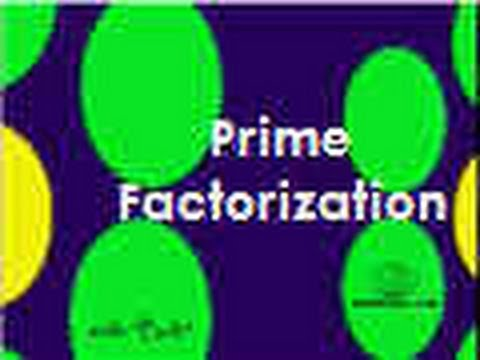## Factors and Factorisation

Subject: Compulsory Maths

#### Overview

The factor which is present in each term is taken as common and each term of the expression is divided by the common factor and the quotient represents the factorization of the expression.

#### Factorisation of expressions which have a common factor in each of its terms

The factor which is present in each term is taken as common and each term of the expression divided by the common factor and the quotient represents the factorization of the expression. For example,

ax+ay

= a(x+y)

= axy2 + ax2y

= 2xy(y + 2x)

Factorisation of expressions having a common factor in the group of terms

In this case, the terms of the given expression are arranged in groups in such a way that each group has a common factor. For example,a2 + ab + ca + bc

a(a+b) + c(a+b)

(a+b)(a+c)

Factorisation of expressions having the difference of two squared terms

The algebraic expressiona2 - b2 is the difference of two squared terms. Here a2 and b2 are the squared terms and a and b are their square roots respectively. We have learnt that a2 - b2 is the product of (a+b) and(a-b)

$\therefore$ a2 - b2 = (a+b) (a-b)

So, (a+b) and (a-b) are the factors of a2 - b2

To factorise such expression, we should re-write given terms in the form of a2 - b2.

Then, a2 - b2 = (a+b) (a-b) represents the factorise. For example,

a) 4x2 + 9y2

= (2x)2 - (3y)2

= (2x+3y)(2x-3y)

b) 9x2 + 25y2

= (3x)2 + (5y)2

= (3x+5y)(3x-5y)

Factorisation of expressions of the form x2 + px + q

While factorising a trimonial expression of the formx2 + px + q, we should search any two number a and b such that a+b = p and ab = q. Clearly a and b must be the factors of q. Then px is expanded in the form ax + bx and factorization is performed by grouping.

##### Things to remember
• The terms of the given expression are arranged in groups in such a way that each group has a common factor.
• The algebraic expression a2 - b2 is the difference of two squared terms.
• It includes every relationship which established among the people.
• There can be more than one community in a society. Community smaller than society.
• It is a network of social relationships which cannot see or touched.
• common interests and common objectives are not necessary for society.
##### Videos for Factors and Factorisation##### Prime Factorization (factor tree)

Solution:

ax + ay

= a(x+y)

Solution:

2px×x -2×3p×px

= 2px(x-3p)

Solution:

3a(x+y) - 4b(x+y)

(x+y) (3a - 4b)

Solution:

a2 + ab + ca + bc

= a(a+b) + c(a+b)

= (a+b) (a+c)

Solution:

x2 - 3a + 3x - ax

= x2+ 3x - ax - 3a

= x(x+3) -a (x+3)

= (x+3) (x-a)

Solution:

= (9x2)2 - (4y2)2

= (9x2 + 4y2) (9x2 - 4y2)

= (9x2 + 4y2) [(3x2)2 - (2y)2]

= (9x2 + 4y2) (3x + 2y) (3x - 2y)

Solution:

Here, (a+b) = 2

$\therefore$ (a+b)3 = 23

or, a3 + 3a2b + 3ab2 + b3 = 8

or, a3 + b3 + 3ab(a+b) = 8

or, a3 + b3 + 3ab×2 = 8

or, a3 + b3 + 6ab = 8

So, the reqiured value of a3 + b3 + 6ab is 8.

Solution:

= 24(40 - 20)

= 24×20

= 480

Solution:

= (65+55) (65-55)

= 120×10

= 1200